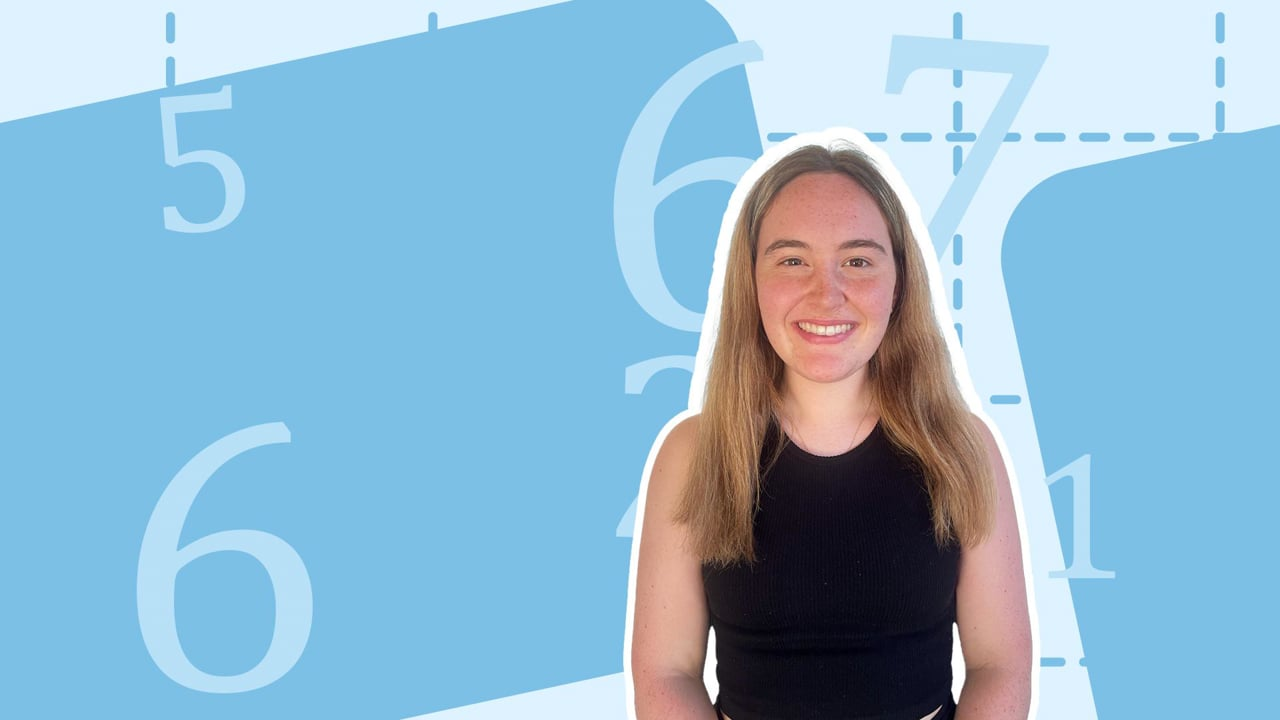Chapter overviewMaths

Exam board

AQA

Number

Algebra

Graphs

Ratio proportion and rates of change

Shapes and area

Angles and geometry

Trigonometry

Probability

Statistics

Maths

# Multiplying and dividing by powers of 100%

Summary

# Multiplying and dividing by powers of 10

## ​​In a nutshell

When multiplying or dividing by powers of $10$​, you will need to move the decimal point and potentially include some extra zeros. If you are multiplying, the decimal point moves towards the right as the number increases in value. If you are dividing, the decimal point moves towards the left as the number decreases in value.

## Multiplying by powers of 10

#### ​​Procedure

 1 Count the number of zeros the 10, 100, 1000 etc. has. 2 Shift the decimal point of the number being multiplied by that many places to the right. If your decimal point reaches the end of the number, it helps to extend the number by writing in extra zeros at the end of the number, as required. 3 The answer is the new number with the new location of the decimal point.

##### Example 1

What is $658.401 \times 10000$?

$10000$ has $4$ zeros, hence move the decimal point $4$ places to the right. As there are not $4$ digits after the decimal point you can write $658.401$ as $658.4010$.

Shifting the decimal point $4$​ places to the right gives $\underline{6584010}$.

## Dividing by powers of 10

#### Procedure

 1 Count the number of zeros the 10, 100, 1000 etc. has. 2 Shift the decimal point of the number being multiplied by that many places to the left. If your decimal point reaches the end of the number, it helps to extend the number by writing in extra zeros at the beginning of the number, as required. 3 The answer is the new number with the new location of the decimal point.  If necessary, you may need to write a zero ahead of the decimal point.

##### Example 2

What is $7304.08\div 100000$?

$100000$ has $5$ zeros, hence move the decimal point $5$ places to the left. As there are not $5$ digits ahead of the decimal point you can write $7304.08$ as $07304.08$

Shifting the decimal point $5$​ places to the left gives $\underline{0.0730408}$.

## Want to find out more? Check out these other lessons!

Multiplying and dividing by powers of 10

FAQs

• Question: Does it matter if you do multiplication or division first?

Answer: Multiplication and division can be done together. In other words, it doesn't matter if you do division or multiplication first, but they must be done after brackets and indices and before addition and subtraction.

• Question: When you're multiplying does the order of the factors matter?

Answer: The commutative property says that the order of the numbers doesn't matter in multiplication.

• Question: What happens when you multiply by 10, 100 or 1000?

Answer: When you multiply whole numbers by 10, 100, 1000, you simply rewrite the numbers and put some extra zeros at the end. If you multiply a whole number by a 10, then you write one zero at the end.

Theory

Exercises